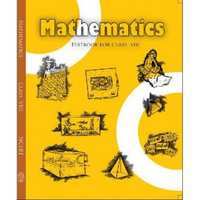Share

NCERT solutions for Class 8 Mathematics chapter 4 - Practical Geometry

Mathematics Textbook for Class 8

NCERT Mathematics Class 8Chapter 4: Practical Geometry

Ex. 4.10Ex. 4.20Ex. 4.30Ex. 4.40Ex. 4.50

Chapter 4: Practical Geometry Exercise 4.10 solutions [Page 60]

Ex. 4.10 | Q 1.1 | Page 60

Construct the following quadrilaterals.

AB = 4.5 cm

BC = 5.5 cm

CD = 4 cm

AD = 6 cm

AC = 7 cm

Ex. 4.10 | Q 1.2 | Page 60

Construct the following quadrilaterals.

JU = 3.5 cm

UM = 4 cm

MP = 5 cm

PJ = 4.5 cm

PU = 6.5 cm

Ex. 4.10 | Q 1.3 | Page 60

Construct the following quadrilaterals.

Parallelogram MORE

OR = 6 cm

RE = 4.5 cm

EO = 7.5 cm

Ex. 4.10 | Q 1.4 | Page 60

Construct the following quadrilaterals.

Rhombus BEST

BE = 4.5 cm

ET = 6 cm

Chapter 4: Practical Geometry Exercise 4.20 solutions [Page 62]

Ex. 4.20 | Q 1.1 | Page 62

Construct the following quadrilaterals.

LI = 4 cm

IF = 3 cm

TL = 2.5 cm

LF = 4.5 cm

IT = 4 cm

Ex. 4.20 | Q 1.2 | Page 62

Construct the following quadrilaterals.

OL = 7.5 cm

GL = 6 cm

GD = 6 cm

LD = 5 cm

OD = 10 cm

Ex. 4.20 | Q 1.3 | Page 62

Construct the following quadrilaterals.

Rhombus BEND

BN = 5.6 cm

DE = 6.5 cm

Chapter 4: Practical Geometry Exercise 4.30 solutions [Page 64]

Ex. 4.30 | Q 1.1 | Page 64

Construct the following quadrilaterals.

MO = 6 cm

OR = 4.5 cm

∠M = 60°

∠O = 105°

∠R = 105°

Ex. 4.30 | Q 1.2 | Page 64

Construct the following quadrilaterals.

PL = 4 cm

LA = 6.5 cm

∠P = 90°

∠A = 110°

∠N = 85°

Ex. 4.30 | Q 1.3 | Page 64

Construct the following quadrilaterals.

Parallelogram HEAR

HE = 5 cm

EA = 6 cm

∠R = 85°

Ex. 4.30 | Q 1.4 | Page 64

Construct the following quadrilaterals.

Rectangle OKAY

OK = 7 cm

KA = 5 cm

Chapter 4: Practical Geometry Exercise 4.40 solutions [Page 67]

Ex. 4.40 | Q 1.1 | Page 67

Construct the following quadrilaterals,

DE = 4 cm

EA = 5 cm

AR = 4.5 cm

∠E = 60°

∠A = 90°

Ex. 4.40 | Q 1.2 | Page 67

Construct the following quadrilaterals,

TR = 3.5 cm

RU = 3 cm

UE = 4 cm

∠R = 75°

∠U = 120°

Chapter 4: Practical Geometry Exercise 4.50 solutions [Page 68]

Ex. 4.50 | Q 1 | Page 68

Draw the following:

The square READ with RE = 5.1 cm

Ex. 4.50 | Q 2 | Page 68

Draw the following:

A rhombus whose diagonals are 5.2 cm and 6.4 cm long.

Ex. 4.50 | Q 3 | Page 68

Draw the following:

A rectangle with adjacent sides of length 5 cm and 4 cm.

Ex. 4.50 | Q 4 | Page 68

Draw the following:

A parallelogram OKAY where OK = 5.5 cm and KA = 4.2 cm.

Chapter 4: Practical Geometry

Ex. 4.10Ex. 4.20Ex. 4.30Ex. 4.40Ex. 4.50

NCERT Mathematics Class 8NCERT solutions for Class 8 Mathematics chapter 4 - Practical Geometry

NCERT solutions for Class 8 Maths chapter 4 (Practical Geometry) include all questions with solution and detail explanation. This will clear students doubts about any question and improve application skills while preparing for board exams. The detailed, step-by-step solutions will help you understand the concepts better and clear your confusions, if any. Shaalaa.com has the CBSE Mathematics Textbook for Class 8 solutions in a manner that help students grasp basic concepts better and faster.

Further, we at Shaalaa.com are providing such solutions so that students can prepare for written exams. NCERT textbook solutions can be a core help for self-study and acts as a perfect self-help guidance for students.

Concepts covered in Class 8 Mathematics chapter 4 Practical Geometry are Introduction of Pratical Geometry, When the Lengths of Four Sides and a Diagonal Are Given, When Two Diagonals and Three Sides Are Given, When Two Adjacent Sides and Three Angles Are Known, When Three Sides and Two Included Angles Are Given, Some Special Cases.

Using NCERT Class 8 solutions Practical Geometry exercise by students are an easy way to prepare for the exams, as they involve solutions arranged chapter-wise also page wise. The questions involved in NCERT Solutions are important questions that can be asked in the final exam. Maximum students of CBSE Class 8 prefer NCERT Textbook Solutions to score more in exam.

Get the free view of chapter 4 Practical Geometry Class 8 extra questions for Maths and can use Shaalaa.com to keep it handy for your exam preparation

S